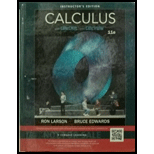Chapter 10, Problem 118RE### Calculus (MindTap Course List)

11th Edition
Ron Larson + 1 other
ISBN: 9781337275347

#### Solutions

Chapter
Section### Calculus (MindTap Course List)

11th Edition
Ron Larson + 1 other
ISBN: 9781337275347
Textbook Problem

# Identifying and Sketching a Conic In Exercises 113-118, find the eccentricity and the distance from the pole to the directrix of the conic. Then identify the conic and sketch its graph. Use a graphing utility to confirm y our results. r = 8 2 − 5 cos θ

To determine

To calculate: The eccentricity and the distance between the pole and the directrix of the conic r=825cosθ. Then identify the type of conic and sketch the graph of the conic r=825cosθ. Use graphing utility to confirm the results.

Explanation

Given:

The polar equation is r=825cosθ.

Calculation:

Consider the equation:

r=825cosθ

The standard equation of a conic with eccentricity e and distance between the pole and the directrix d is:

r=ed1+ecosθ

Multiply and divide the fraction by 2. Then,

r=8225cosθ2

So,

r=4152cosθ

Compare the given equation with the standard equation r=ed1+ecosθ, ed=4 and e=52

### Still sussing out bartleby?

Check out a sample textbook solution.

See a sample solution

#### The Solution to Your Study Problems

Bartleby provides explanations to thousands of textbook problems written by our experts, many with advanced degrees!

Get Started

## Additional Math Solutions

#### Factoring Factor the expression completely. 31. x6 1

Precalculus: Mathematics for Calculus (Standalone Book)

#### Describe the set of points (x,y) such that x2+y2=0.

Finite Mathematics and Applied Calculus (MindTap Course List)

#### In Problems 27 – 30, solve for y in terms of x. 30.

Mathematical Applications for the Management, Life, and Social Sciences